# ISC Class 12 Chemistry Question Paper Solution 2018

The ISC Class 12 Chemistry – Paper I (Theory) was conducted on 5th March 2018. The exam started at 2 PM. The paper was of 70 marks and 3 hours of time duration. Here, students can find the answers to all questions in the form of ISC Class 12 Chemistry Question Paper Solution 2018. The answers are provided in the same format as expected to be written by a student during the exam. We have also provided the step marking for each answer so that students can understand how marks are awarded in the board exam. They can download, both the ISC Class 12 Chemistry Question Paper and Solution 2018 Pdf from the link below.

Students can also access the Solved ISC Class 12 Chemistry Previous Year Question Papers compiled at one place. They can have a look at the ISC Class 12 Chemistry Question Paper Solution 2018 below.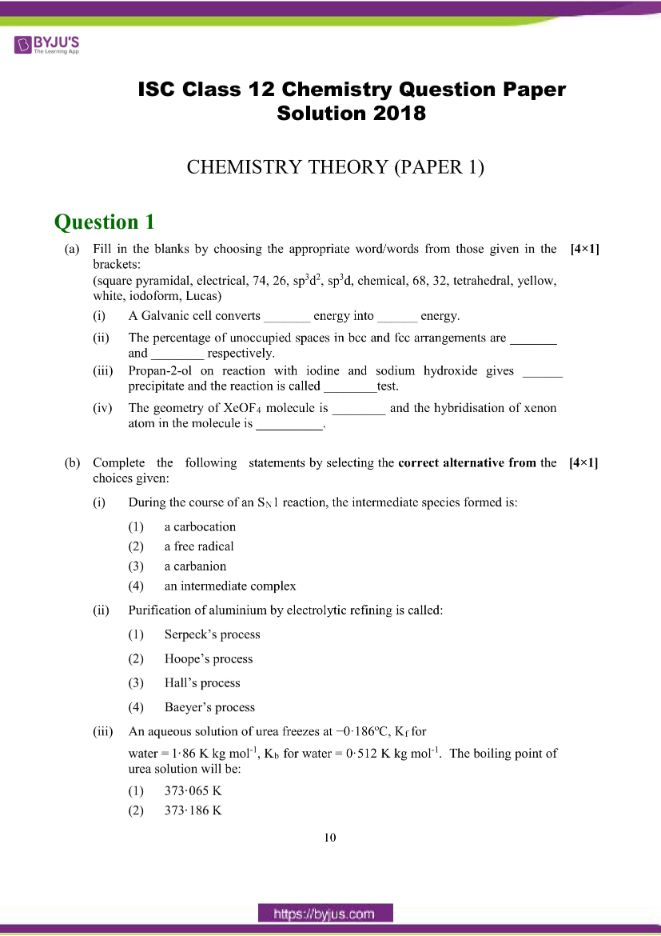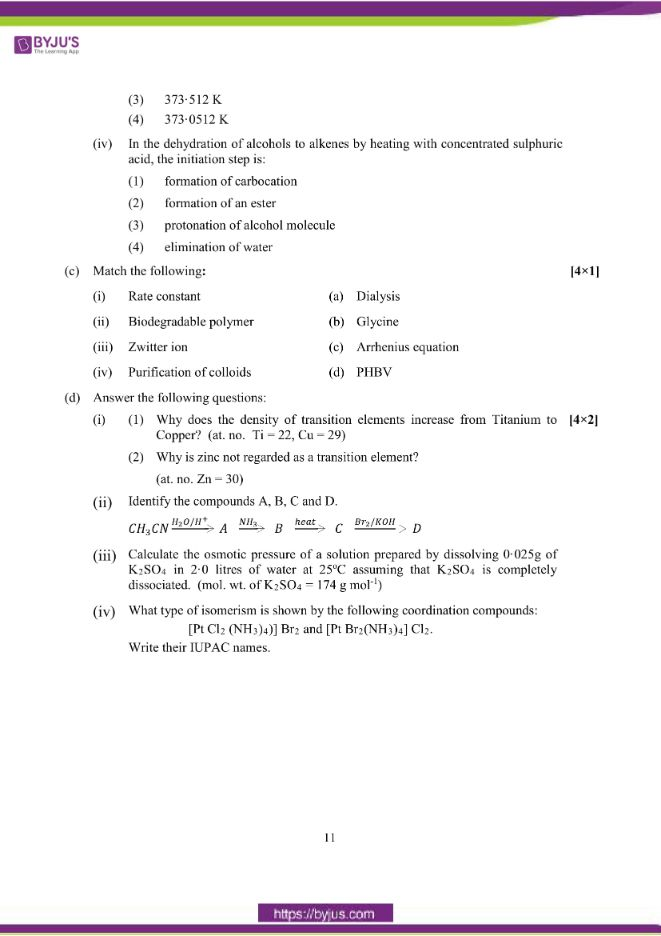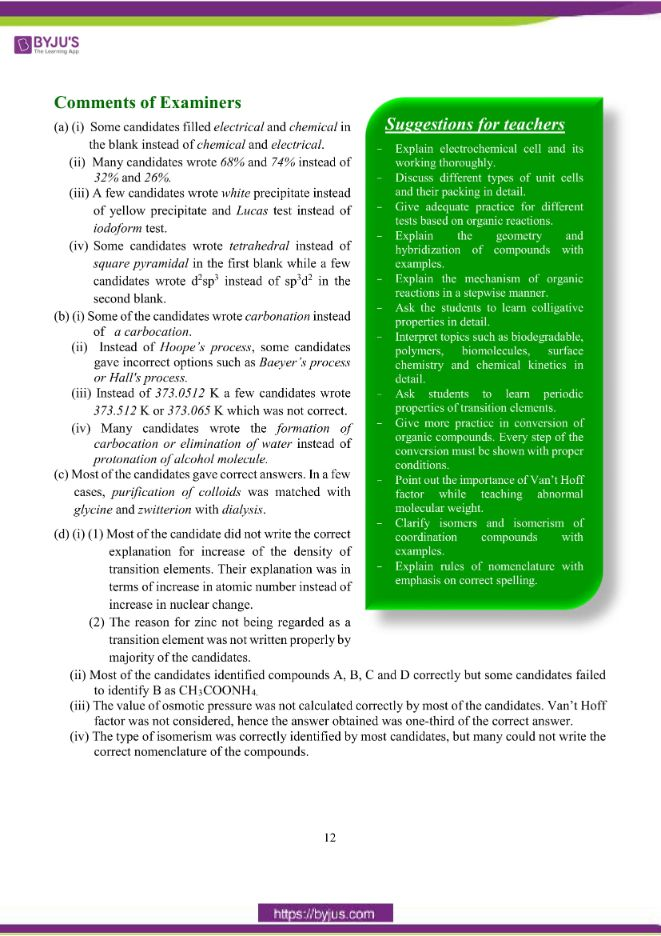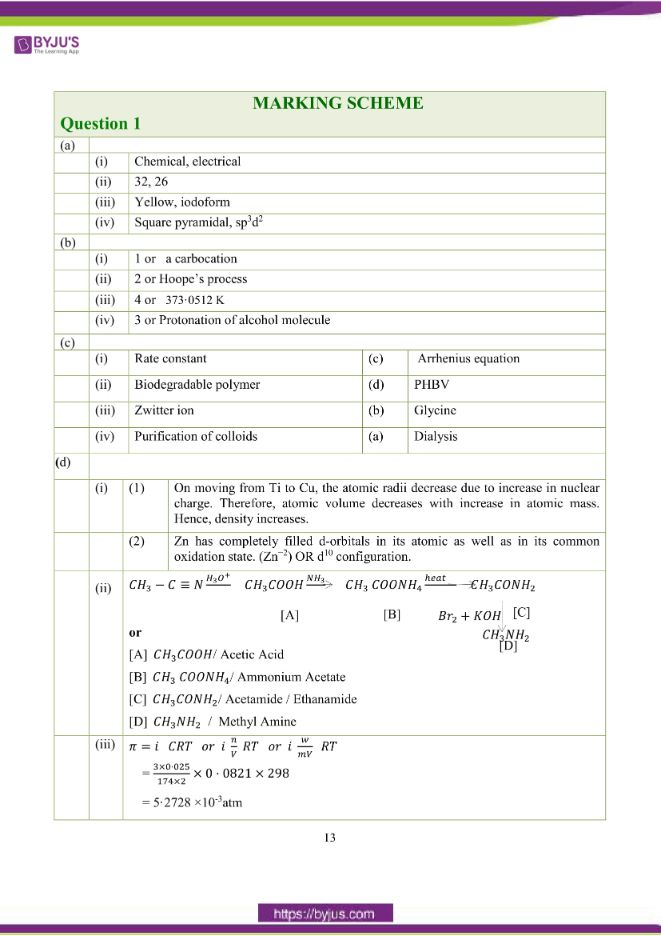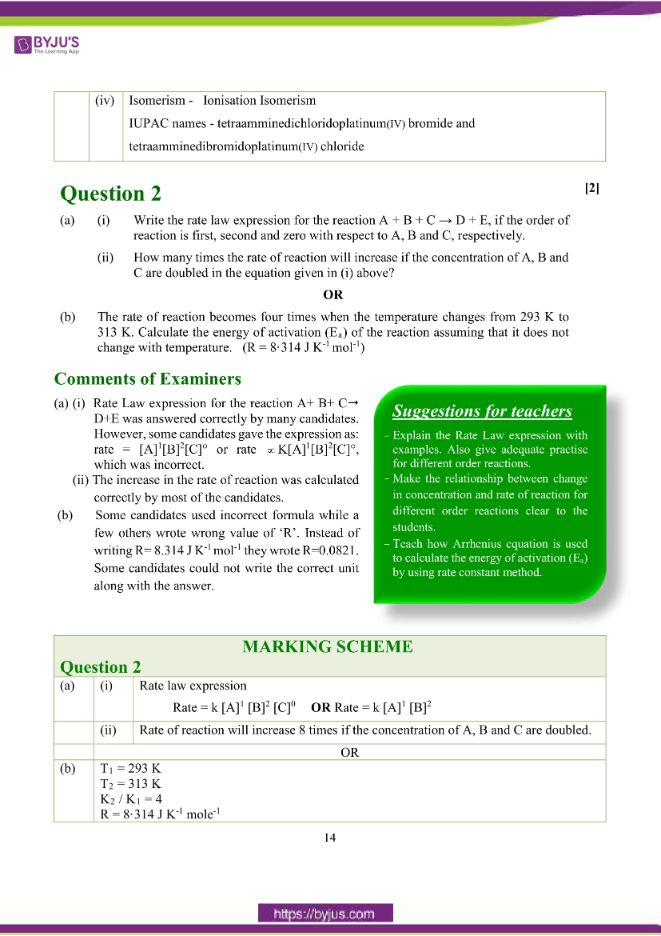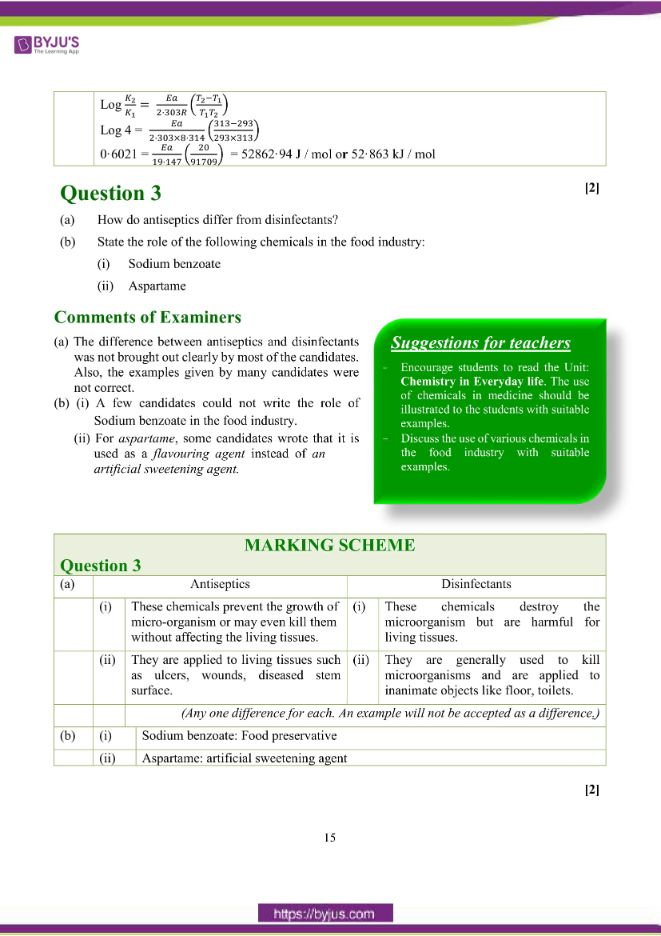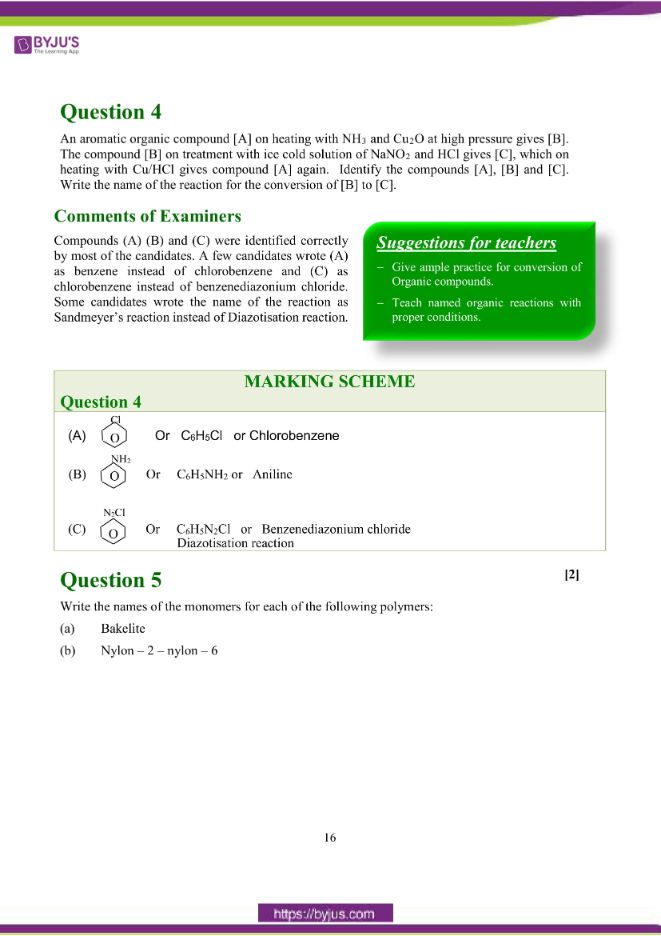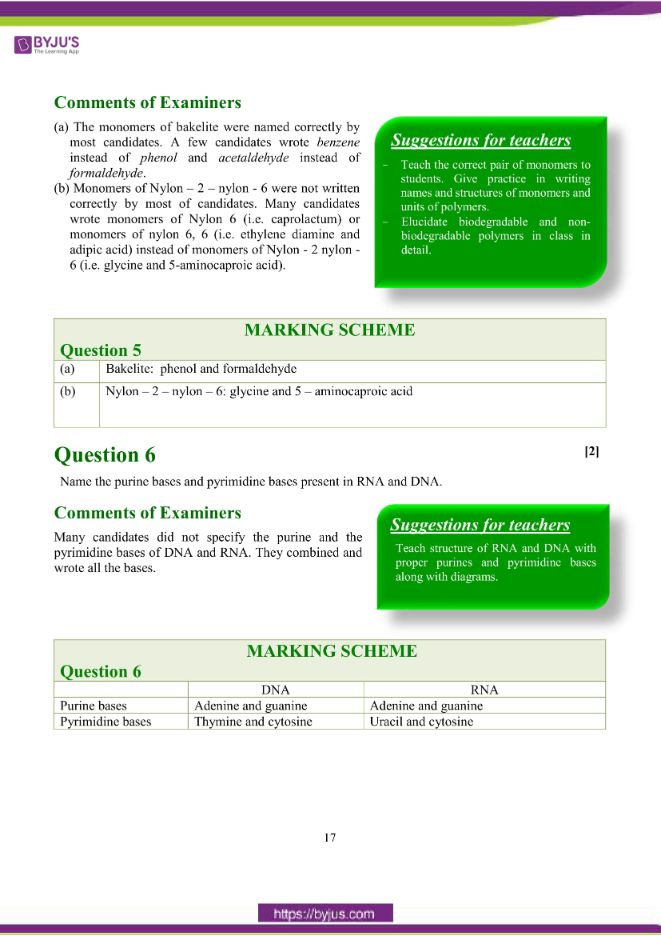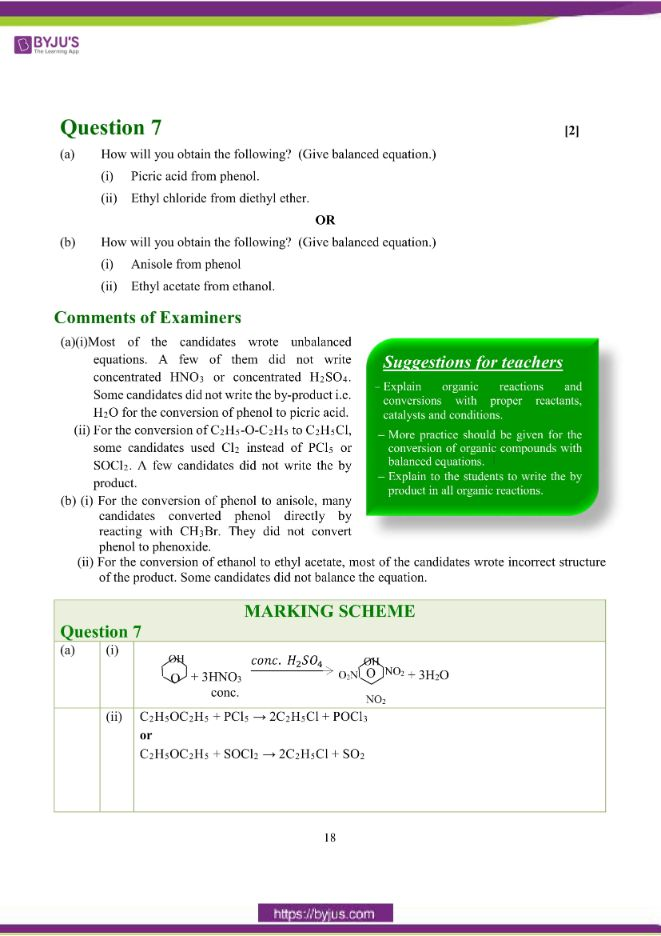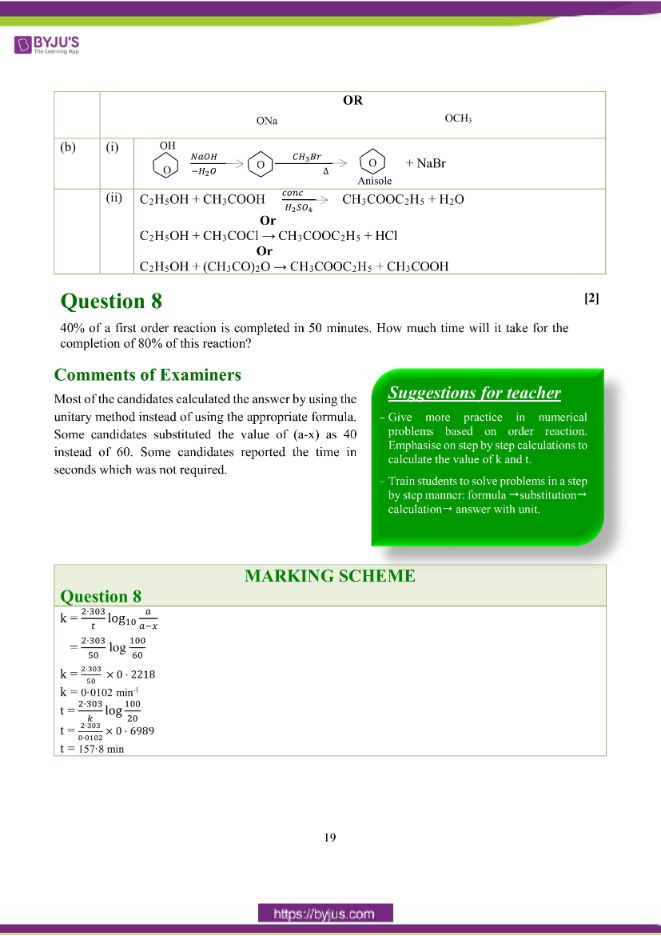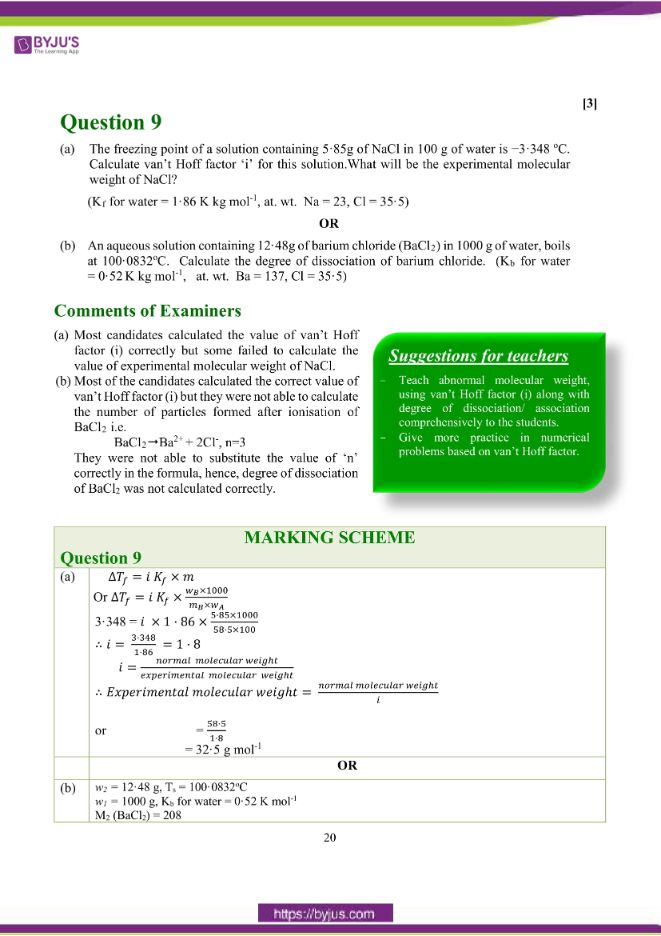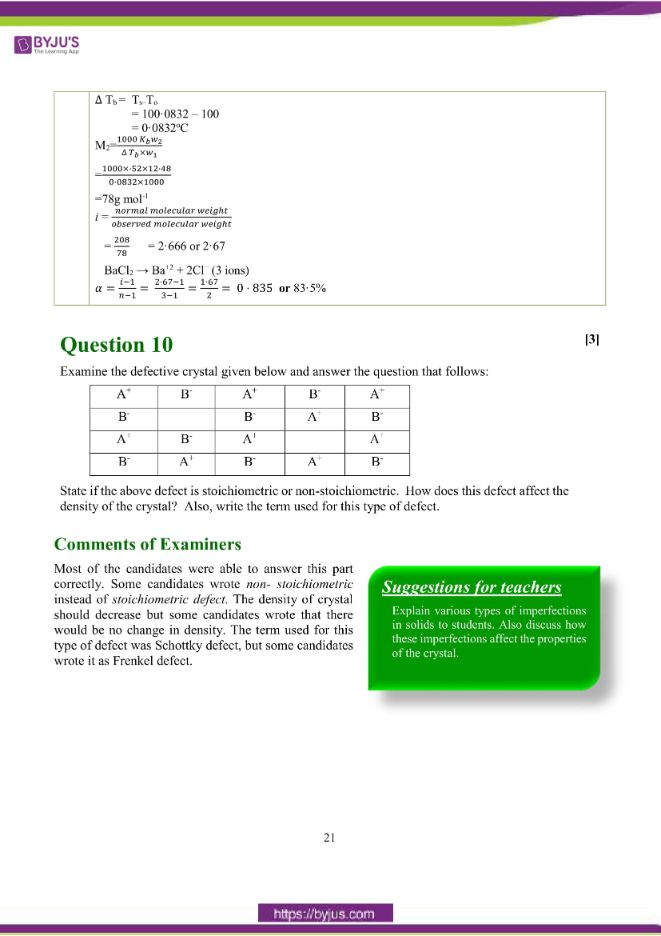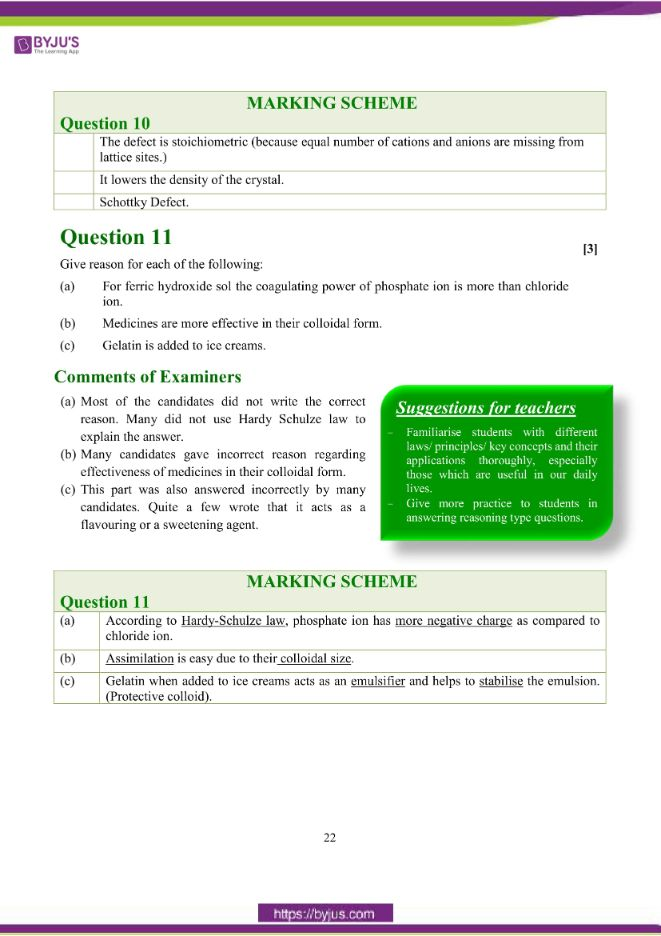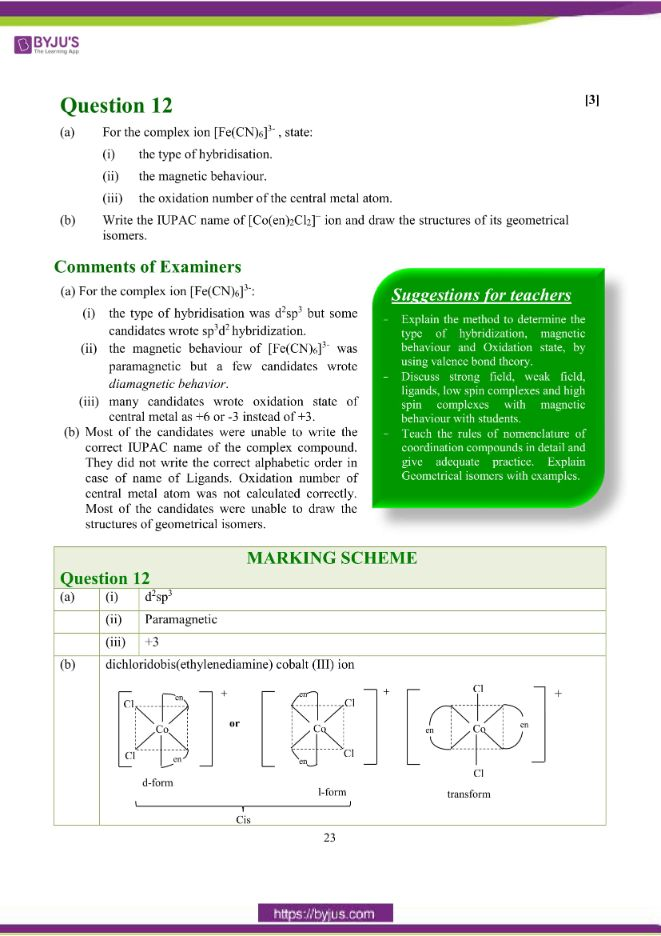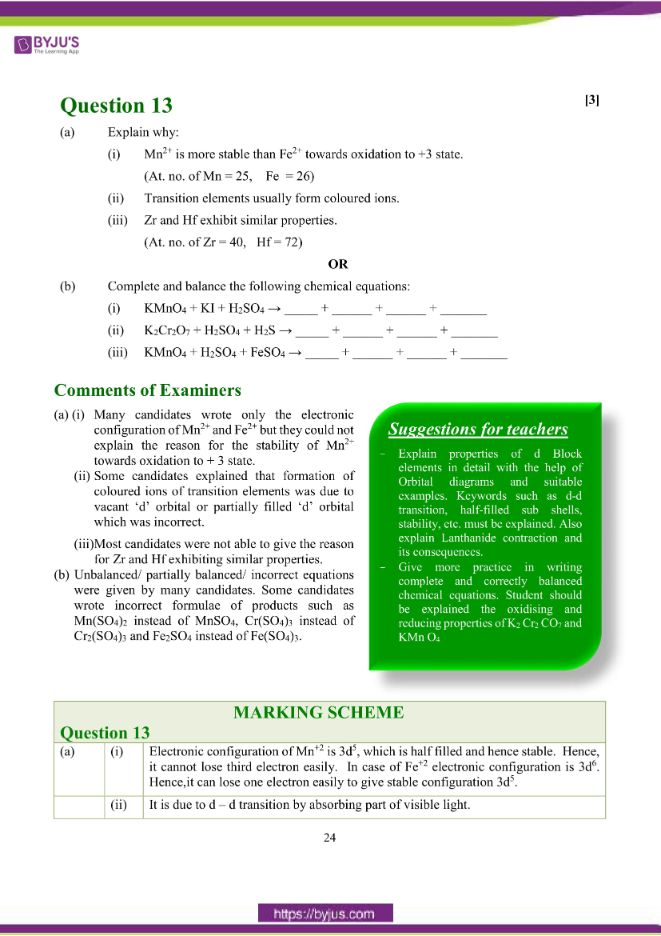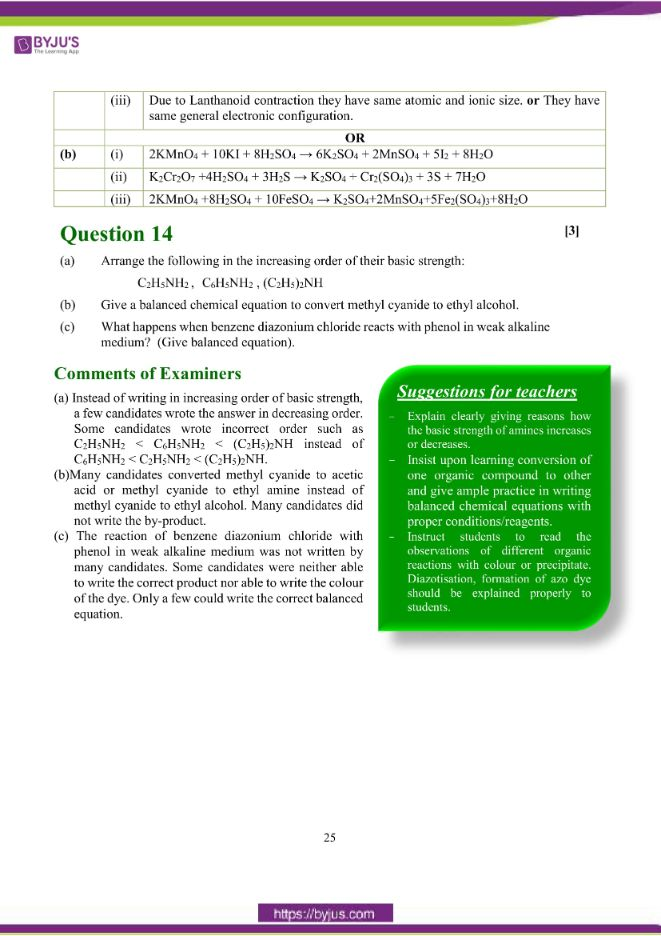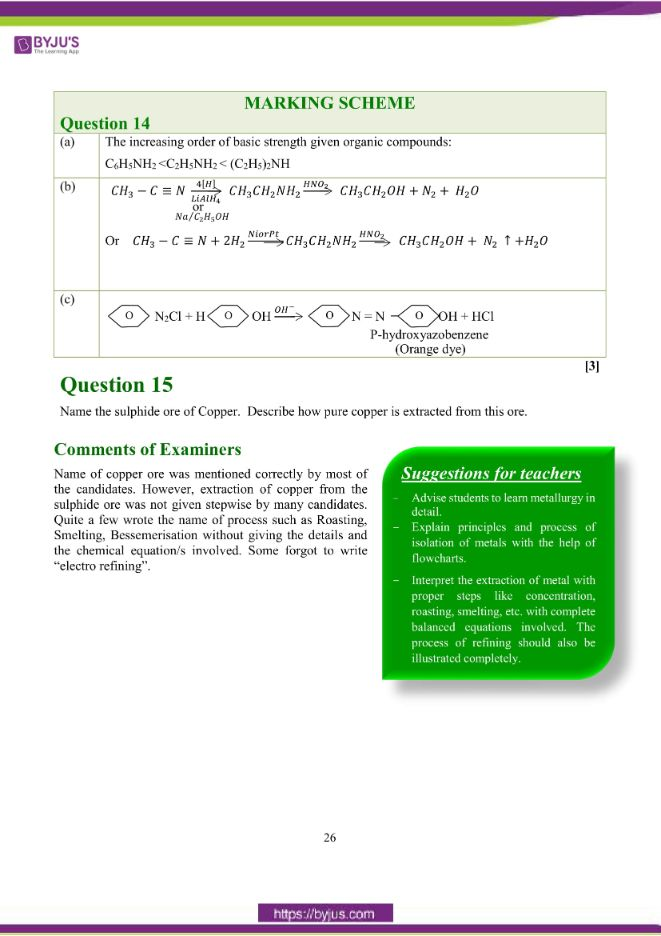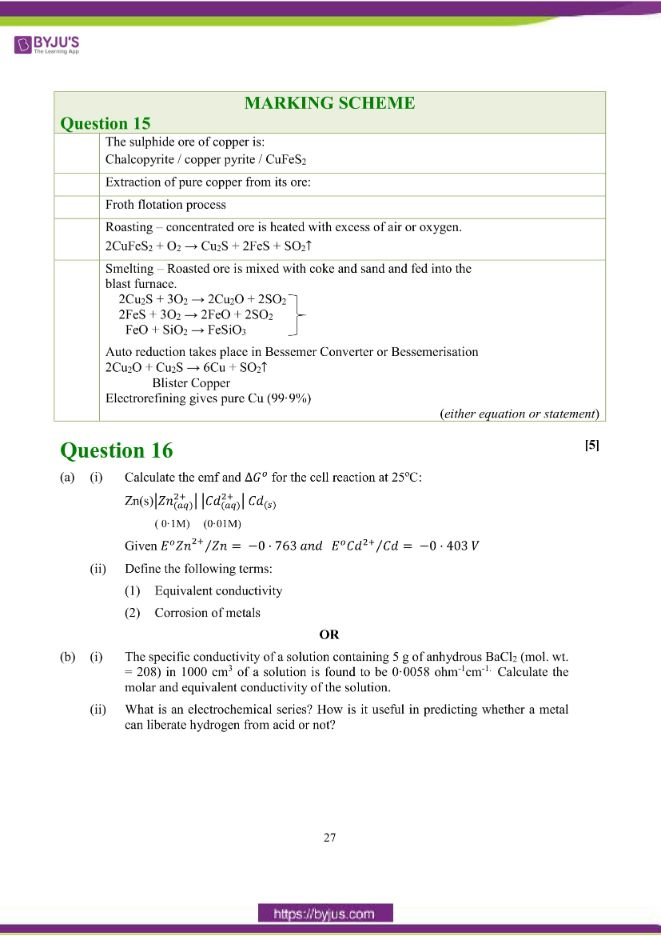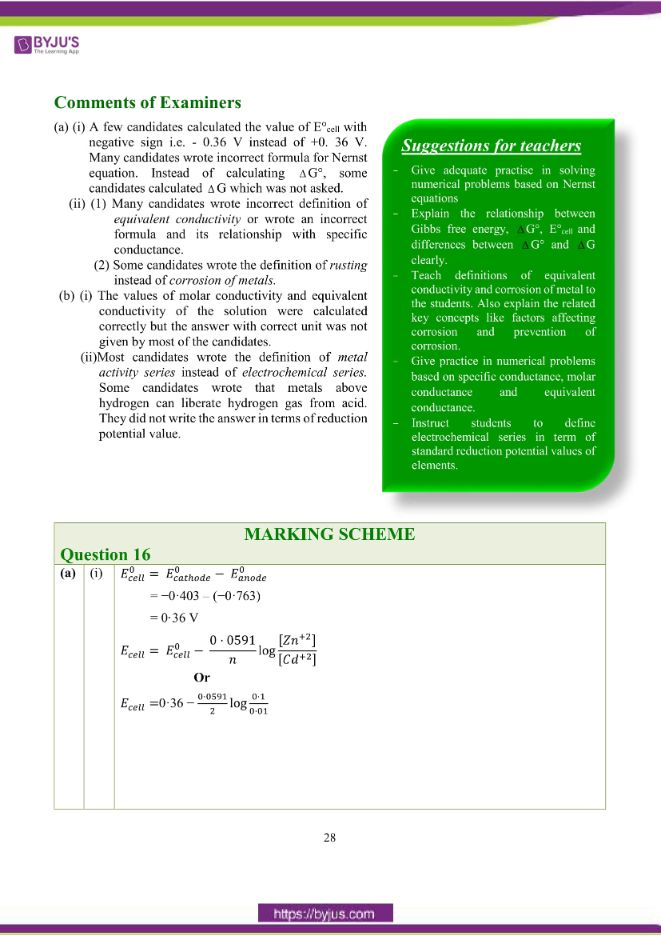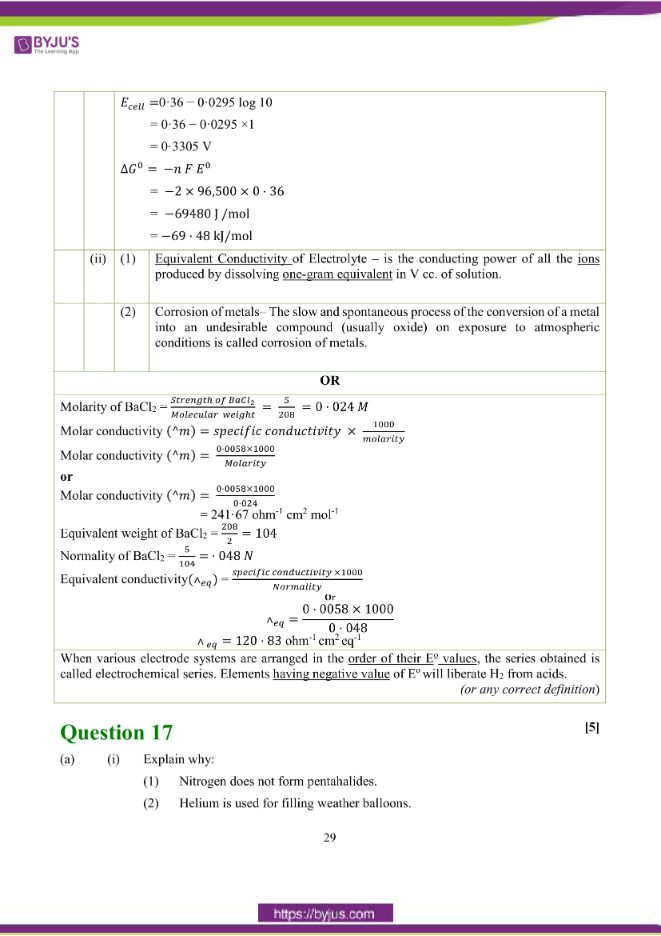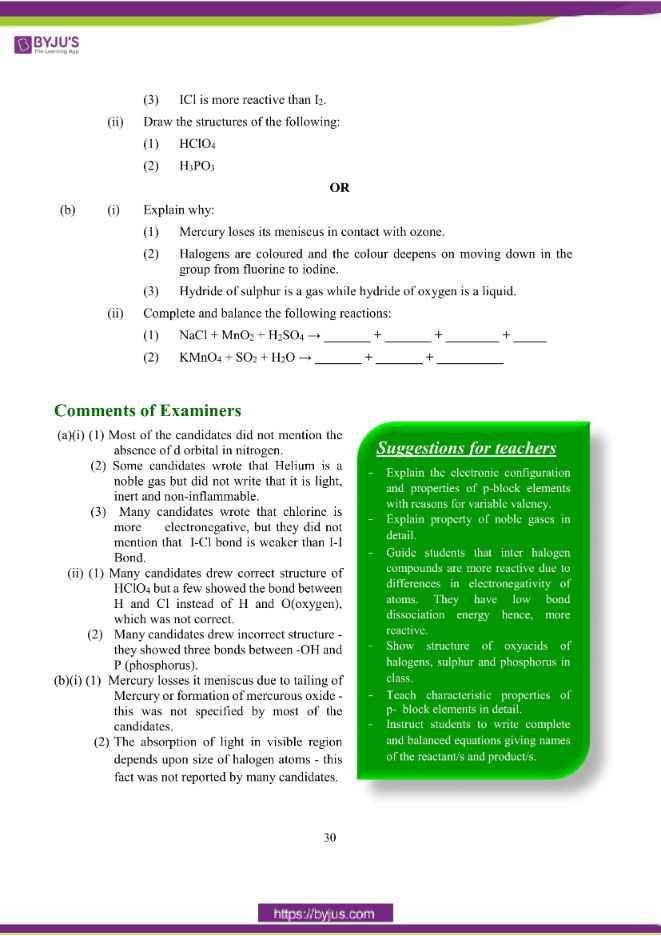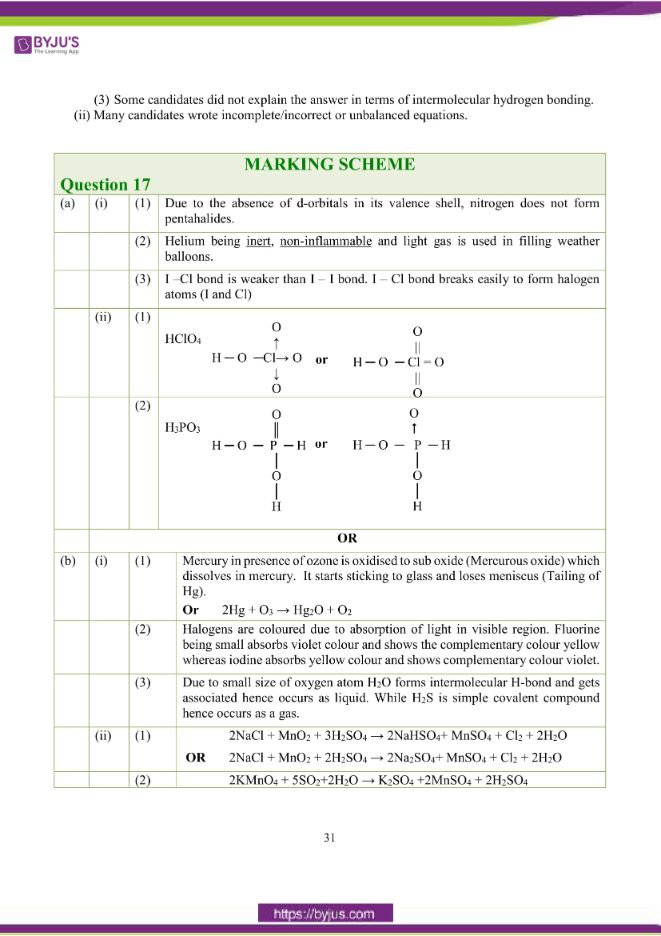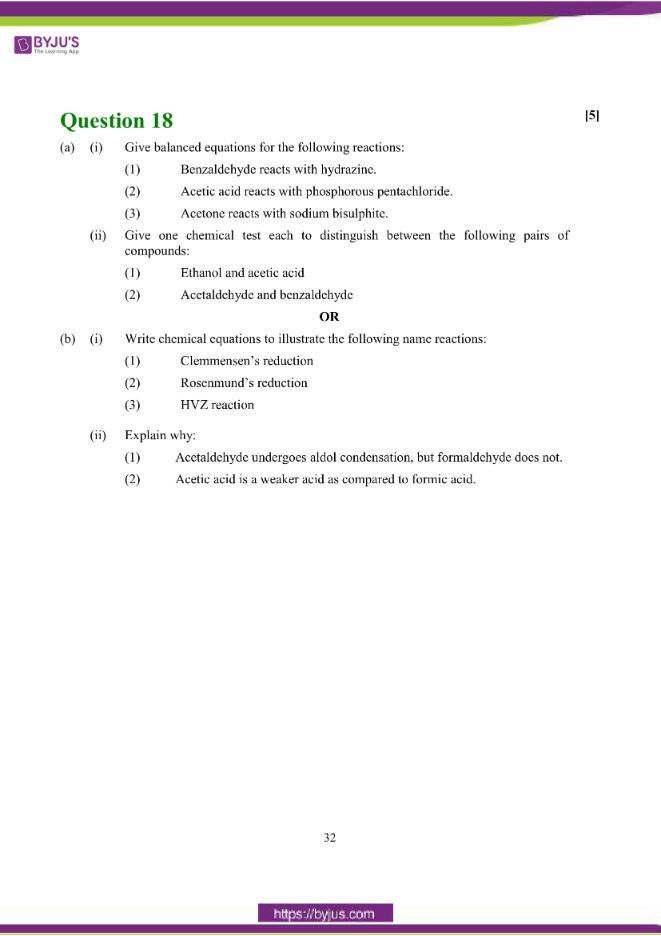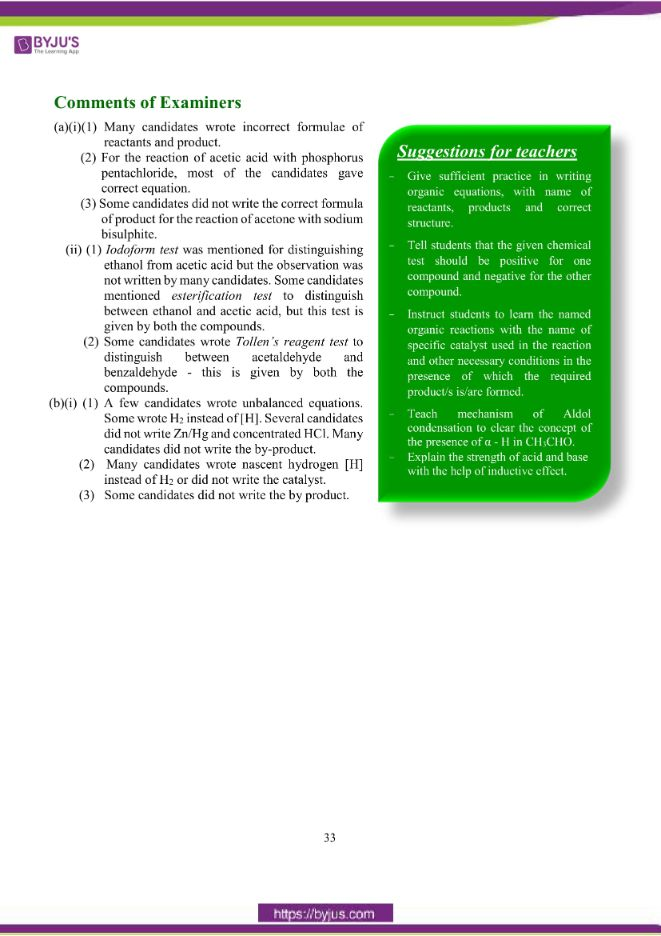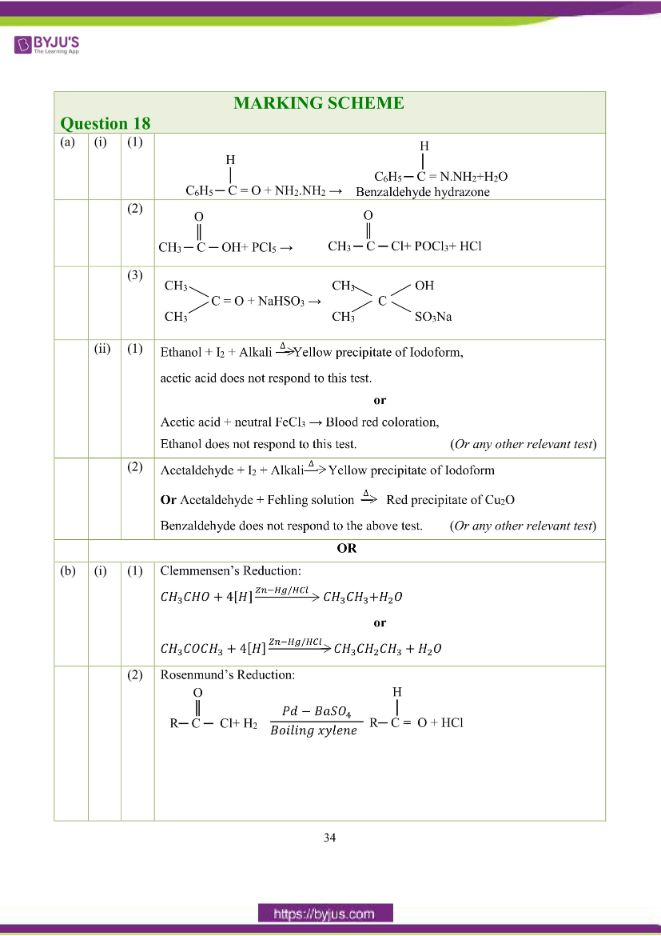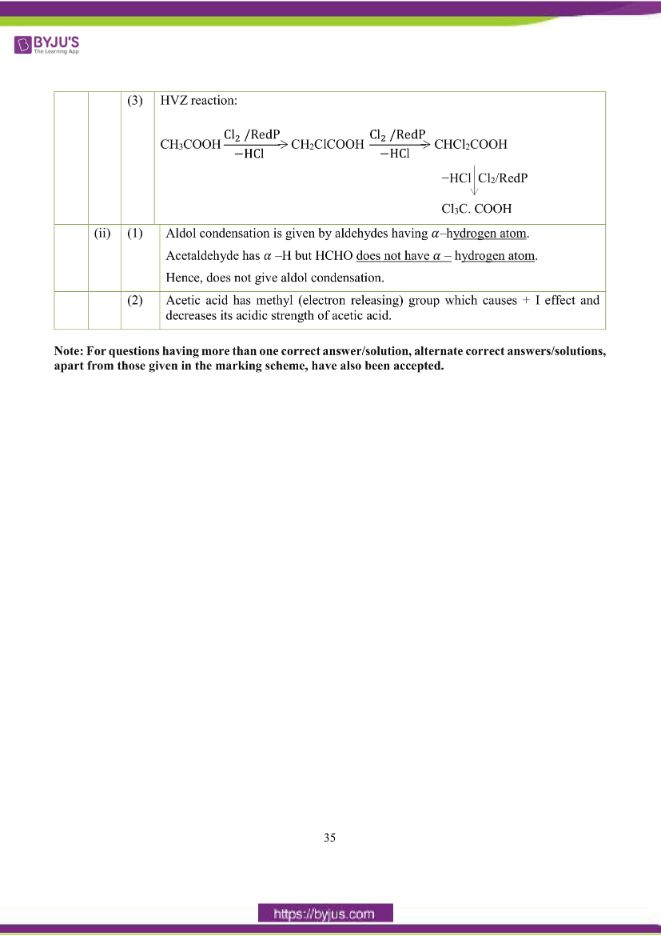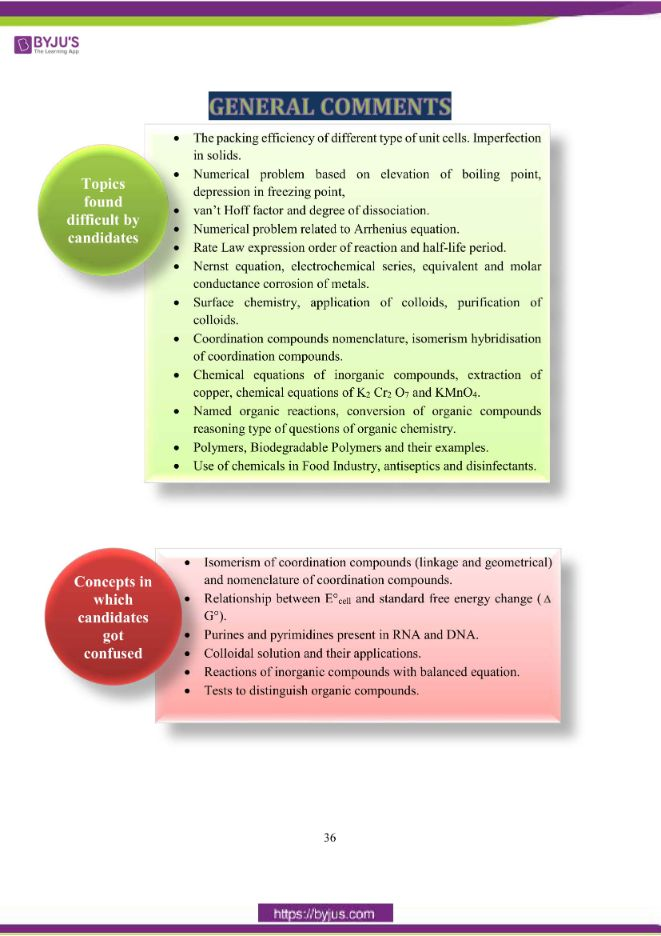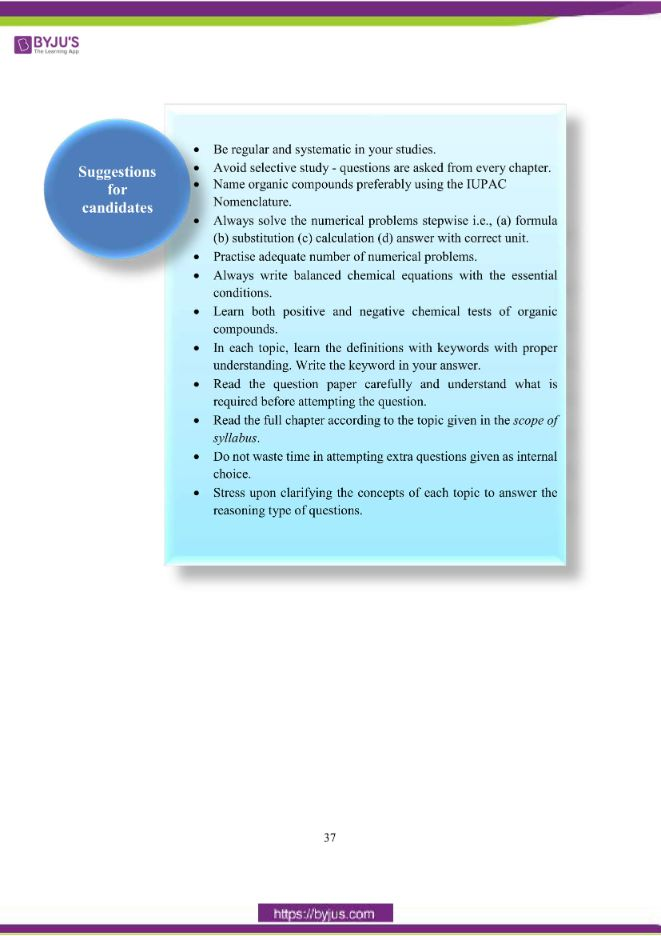### Difficult Topics of ISC Class 12 Chemistry Paper 2018

Topics which students found difficult while solving the Chemistry 2018 paper are:

• The packing efficiency of different types of unit cells. Imperfection in solids.
• Numerical problem based on elevation of boiling point,depression in freezing point,
• van’t Hoff factor and degree of dissociation.
• Numerical problem related to Arrhenius equation.
• Rate Law expression order of reaction and half-life period.
• Nernst equation, electrochemical series, equivalent and molar conductance corrosion of metals.
• Surface chemistry, application of colloids, purification of colloids.
• Coordination compounds nomenclature, isomerism hybridisation of coordination compounds.
• Chemical equations of inorganic compounds, extraction of copper, chemical equations of K2Cr2O7 and KMnO4.
• Named organic reactions, conversion of organic compounds reasoning type of questions of organic chemistry.
• Polymers, Biodegradable Polymers and their examples.
• Use of chemicals in the Food Industry, antiseptics and disinfectants.

### Confusing ISC Class 12 Chemistry Questions 2018

Chemistry concepts on which students got confused are:

• Isomerism of coordination compounds (linkage and geometrical) and nomenclature of coordination compounds.
• Relationship between E°cell and standard free energy change (G°).
• Purines and pyrimidines present in RNA and DNA.
• Colloidal solution and their applications.
• Reactions of inorganic compounds with balanced equations.
• Tests to distinguish organic compounds.

## ISC Class 12 Chemistry Question Paper Solution 2018

### Question 1:

(a) Fill in the blanks by choosing the appropriate word/words from those given in the

brackets:

(square pyramidal, electrical, 74, 26, sp3d2, sp3d, chemical, 68, 32, tetrahedral, yellow, white, iodoform, Lucas)

(i) A Galvanic cell converts _______ energy into ______ energy.

(ii) The percentage of unoccupied spaces in bcc and fcc arrangements are _______ and ________ respectively.

(iii) Propan-2-ol on reaction with iodine and sodium hydroxide gives ______ precipitate and the reaction is called ________test.

(iv) The geometry of XeOF4 molecule is ________ and the hybridisation of the xenon atom in the molecule is __________.

(b) Complete the following statements by selecting the correct alternative from the choices given:

(i) During the course of an SN1 reaction, the intermediate species formed is:

1. a carbocation
3. a carbanion
4. an intermediate complex

(ii) Purification of aluminium by electrolytic refining is called:

1. Serpeck’s process
2. Hoope’s process
3. Hall’s process
4. Baeyer’s process

(iii) An aqueous solution of urea freezes at −0·186oC, Kf for water = 1·86 K kg mol-1, Kb for water = 0·512 K kg mol-1. The boiling point of

urea solution will be:

1. 373·065 K
2. 373·186 K
3. 373·512 K
4. 373·0512 K

(iv) In the dehydration of alcohols to alkenes by heating with concentrated sulphuric acid, the initiation step is:

1. formation of carbocation
2. formation of an ester
3. protonation of alcohol molecule
4. elimination of water

(c) Match the following:

 (i) Rate constant (a) Dialysis (ii) Biodegradable polymer (b) Glycine (iii) Zwitter ion (c) Arrhenius equation (iv) Purification of colloids (d) PHBV

(i)

1. Why does the density of transition elements increase from Titanium to Copper? (at. no. Ti = 22, Cu = 29)
2. Why is zinc not regarded as a transition element? (at. no. Zn = 30)

(ii) Identify the compounds A, B, C and D.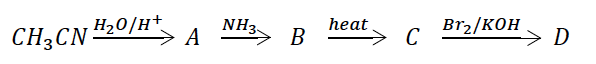(iii) Calculate the osmotic pressure of a solution prepared by dissolving 0·025g of K2SO4 in 2·0 litres of water at 25oC assuming that K2SO4 is completely dissociated. (mol. wt. of K2SO4 = 174 g mol-1)

(iv) What type of isomerism is shown by the following coordination compounds:

[Pt Cl2 (NH3)4)] Br2 and [Pt Br2(NH3)4] Cl2.

Write their IUPAC names.

(ii) 32, 26

(iii) Yellow, iodoform

(iv) Square pyramidal, sp3d2

(b) (i) 1 or a carbocation

(ii) 2 or Hoope’s process

(iii) 4 or 373·0512 K

(iv) 3 or Protonation of alcohol molecule

(c)

 (i) Rate constant (c) Arrhenius equation (ii) Biodegradable polymer (d) PHBV (iii) Zwitter ion (b) Glycine (iv) Purification of colloids (a) Dialysis

(d) (i)

1. On moving from Ti to Cu, the atomic radii decrease due to increase in nuclear charge. Therefore, atomic volume decreases with increase in atomic mass.

Hence, density increases.

1. Zn has completely filled d-orbitals in its atomic as well as in its common oxidation state. (Zn+2) OR d10 configuration.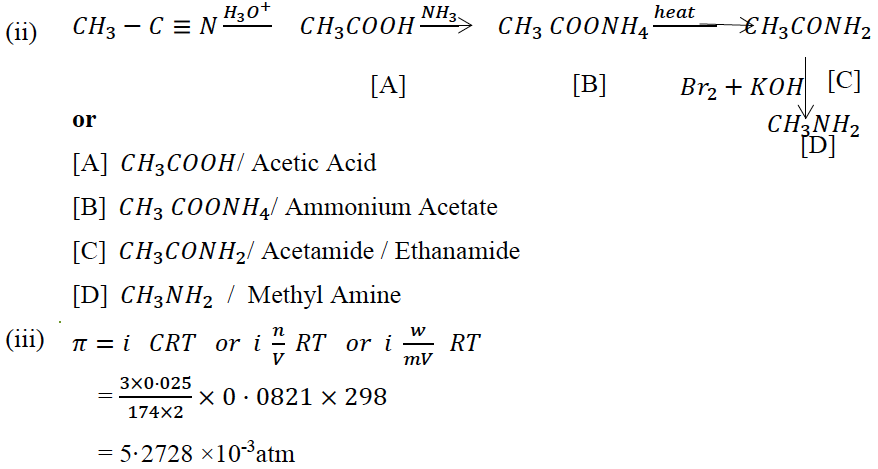(iv) Isomerism – Ionisation Isomerism

IUPAC names – tetraamminedichloridoplatinum(IV) bromide and tetraamminedibromidoplatinum(IV) chloride

### Question 2:

(a) (i) Write the rate law expression for the reaction A + B + C → D + E, if the order of reaction is first, second and zero with respect to A, B and C, respectively.

(ii) How many times the rate of reaction will increase if the concentration of A, B and C are doubled in the equation given in (i) above?

OR

(b) The rate of reaction becomes four times when the temperature changes from 293 K to 313 K. Calculate the energy of activation (Ea) of the reaction assuming that it does not change with temperature. (R = 8·314 J K-1 mol-1)

(a) (i) Rate law expression

Rate = k [A]1 [B]2 [C]0 OR Rate = k [A]1 [B]2

(ii) Rate of reaction will increase 8 times if the concentration of A, B and C are doubled.

OR

(b) T1 = 293 K

T2 = 313 K

K2 / K1 = 4

R = 8.314 J K-1 mole-1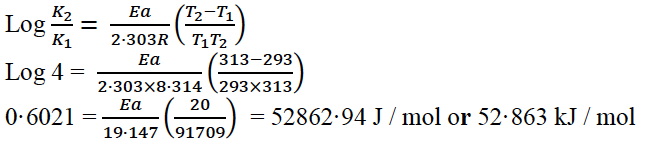### Question 3:

(a) How do antiseptics differ from disinfectants?

(b) State the role of the following chemicals in the food industry:

(i) Sodium benzoate

(ii) Aspartame

 Antiseptics Disinfectants These chemicals prevent the growth of micro-organism or may even kill them without affecting the living tissues. These chemicals destroy the microorganism but are harmful for living tissues. They are applied to living tissues such as ulcers, wounds, diseased stem surface. They are generally used to kill microorganisms and are applied to inanimate objects like floor, toilets.

(b) (i) Sodium benzoate: Food preservative

(ii) Aspartame: artificial sweetening agent

### Question 4: An aromatic organic compound [A] on heating with NH3 and Cu2O at high pressure gives [B]. The compound [B] on treatment with ice cold solution of NaNO2 and HCl gives [C], which on heating with Cu/HCl gives compound [A] again. Identify the compounds [A], [B] and [C]. Write the name of the reaction for the conversion of [B] to [C].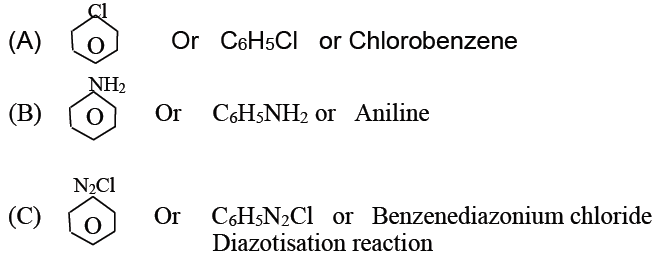### Question 5: Write the names of the monomers for each of the following polymers:

(a) Bakelite

(b) Nylon – 2 – nylon – 6

Answer: (a) Bakelite: phenol and formaldehyde

(b) Nylon – 2 – nylon – 6: glycine and 5 – aminocaproic acid

### Question 6: Name the purine bases and pyrimidine bases present in RNA and DNA.

 DNA RNA Purine bases Adenine and guanine Adenine and guanine Pyrimidine bases Thymine and cytosine Uracil and cytosine

### Question 7:

(a) How will you obtain the following? (Give a balanced equation.)

(i) Picric acid from phenol.

(ii) Ethyl chloride from diethyl ether.

OR

(b) How will you obtain the following? (Give a balanced equation.)

(i) Anisole from phenol

(ii) Ethyl acetate from ethanol.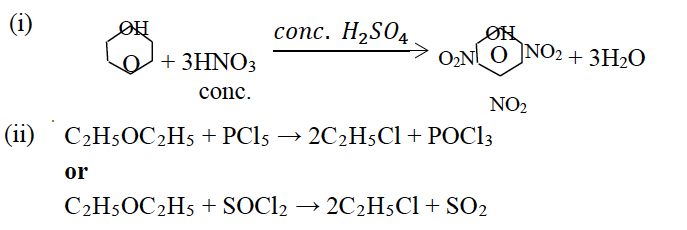(b)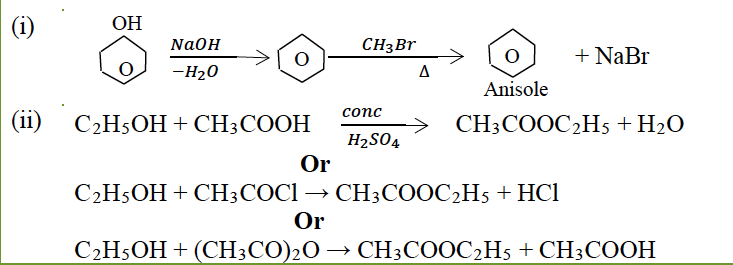### Question 8: 40% of a first order reaction is completed in 50 minutes. How much time will it take for the completion of 80% of this reaction?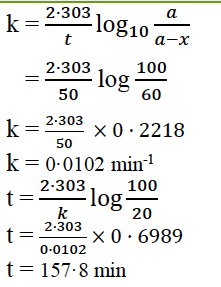### Question 9:

(a) The freezing point of a solution containing 5·85g of NaCl in 100 g of water is −3·348 oC.

Calculate van’t Hoff factor ‘i’ for this solution. What will be the experimental molecular weight of NaCl?

(Kf for water = 1·86 K kg mol-1, at. wt. Na = 23, Cl = 35·5)

OR

(b) An aqueous solution containing 12·48g of barium chloride (BaCl2) in 1000 g of water, boils

at 100·0832oC. Calculate the degree of dissociation of barium chloride. (Kb for water = 0·52 K kg mol-1, at. wt. Ba = 137, Cl = 35·5)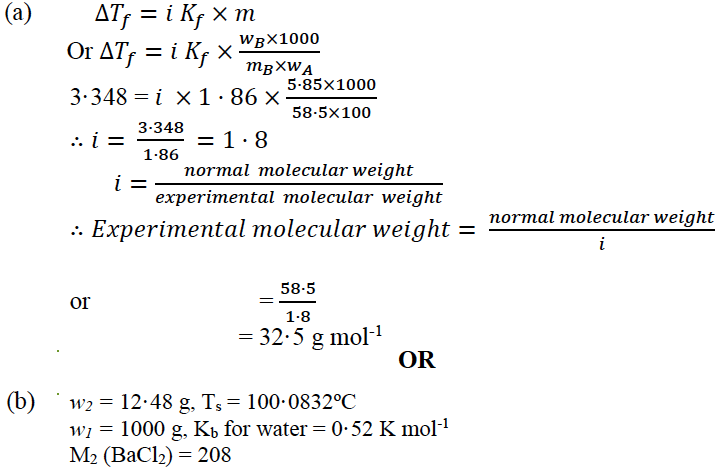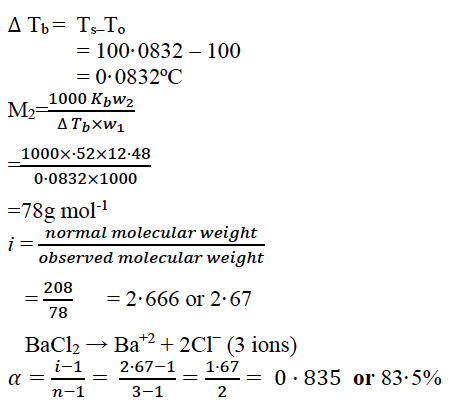### Question 10: Examine the defective crystal given below and answer the question that follows:

 A+ B– A+ B– A+ B– B– A+ B– A+ B– A+ A+ B– A+ B– A+ B–

State if the above defect is stoichiometric or non-stoichiometric. How does this defect affect the density of the crystal? Also, write the term used for this type of defect.

Answer: The defect is stoichiometric (because equal numbers of cations and anions are missing from lattice sites.)

It lowers the density of the crystal.

Schottky Defect.

### Question 11: Give reason for each of the following:

(a) For ferric hydroxide sol the coagulating power of phosphate ion is more than chloride ion.

(b) Medicines are more effective in their colloidal form.

(c) Gelatin is added to ice creams.

(a) According to Hardy-Schulze law, phosphate ions have more negative charge as compared to chloride ions.

(b) Assimilation is easy due to their colloidal size.

(c) Gelatin when added to ice creams acts as an emulsifier and helps to stabilise the emulsion. (Protective colloid).

### Question 12:

(a) For the complex ion [Fe(CN)6]3- , state:

(i) the type of hybridisation.

(ii) the magnetic behaviour.

(iii) the oxidation number of the central metal atom.

(b) Write the IUPAC name of [Co(en)2Cl2]+ ion and draw the structures of its geometrical isomers.

(ii) Paramagnetic

(iii) +3

(b) dichloridobis(ethylenediamine) cobalt (III) ion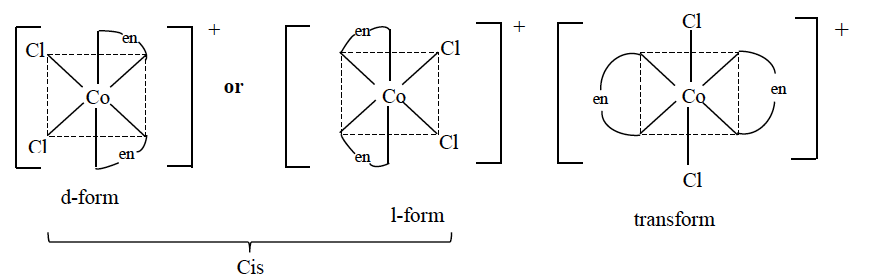### Question 13:

(a) Explain why:

(i) Mn2+ is more stable than Fe2+ towards oxidation to +3 state.

(At. no. of Mn = 25, Fe = 26)

(ii) Transition elements usually form coloured ions.

(iii) Zr and Hf exhibit similar properties.

(At. no. of Zr = 40, Hf = 72)

OR

(b) Complete and balance the following chemical equations:

(i) KMnO4 + KI + H2SO4 → _____ + ______ + ______ + _______

(ii) K2Cr2O7 + H2SO4 + H2S → _____ + ______ + ______ + _______

(iii) KMnO4 + H2SO4 + FeSO4 → _____ + ______ + ______ + _______

Answer: (a) (i) Electronic configuration of Mn+2 is 3d5, which is half filled and hence stable. Hence, it cannot lose a third electron easily. In case of Fe+2 electronic configuration is 3d6. Hence, it can lose one electron easily to give stable configuration 3d5.

(ii) It is due to d – d transition by absorbing part of visible light.

(iii) Due to Lanthanoid contraction they have the same atomic and ionic size. or They have

same general electronic configuration.

OR

(b) (i) 2KMnO4 + 10KI + 8H2SO4 → 6K2SO4 + 2MnSO4 + 5I2 + 8H2O

(ii) K2Cr2O7 +4H2SO4 + 3H2S → K2SO4 + Cr2(SO4)3 + 3S + 7H2O

(iii) 2KMnO4 +8H2SO4 + 10FeSO4 → K2SO4 + 2MnSO4 + 5Fe2(SO4)3 + 8H2O

### Question 14:

(a) Arrange the following in the increasing order of their basic strength:

C2H5NH2 , C6H5NH2 , (C2H5)2NH

(b) Give a balanced chemical equation to convert methyl cyanide to ethyl alcohol.

(c) What happens when benzene diazonium chloride reacts with phenol in a weak alkaline medium? (Give a balanced equation).

Answer: (a) The increasing order of basic strength given organic compounds:

C6H5NH2 < C2H5NH2< (C2H5)2NH

(b)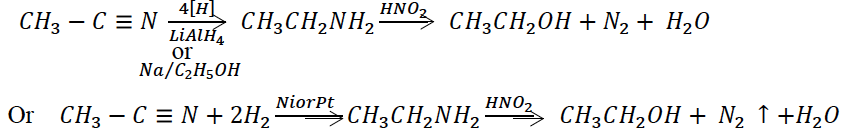(c)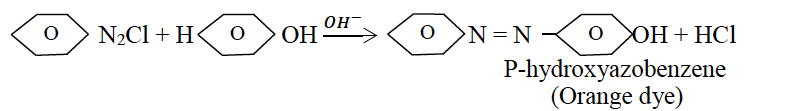### Question 15: Name the sulphide ore of Copper. Describe how pure copper is extracted from this ore.

Answer: The sulphide ore of copper is:

Chalcopyrite / copper pyrite / CuFeS2

Extraction of pure copper from its ore:

Froth flotation process

Roasting – concentrated ore is heated with excess of air or oxygen.

2CuFeS2 + O2 → Cu2S + 2FeS + SO2

Smelting – Roasted ore is mixed with coke and sand and fed into the blast furnace.

2Cu2S + 3O2 → 2Cu2O + 2SO2

2FeS + 3O2 → 2FeO + 2SO2

FeO + SiO2 → FeSiO3

Auto reduction takes place in Bessemer Converter or Bessemerisation

2Cu2O + Cu2S → 6Cu + SO2

Blister Copper

Electrorefining gives pure Cu (99·9%)

### Question 16:

(a) ( i ) Calculate the emf and Δ𝐺0 for the cell reaction at 25oC:

Zn(s) | Zn2+(aq) ( 0·1M) || Cd2+(aq) (0·01M) | Cd(s)

Given 𝐸o 𝑍n2+ ⁄ 𝑍n = −0.763 and 𝐸o 𝐶d2+ ⁄ 𝐶d = −0.403 𝑉

(ii) Define the following terms:

1. Equivalent conductivity
2. Corrosion of metals

OR

(b) (i) The specific conductivity of a solution containing 5 g of anhydrous BaCl2 (mol. wt. = 208) in 1000 cm3 of a solution is found to be 0·0058 ohm-1cm-1. Calculate the molar and equivalent conductivity of the solution.

(ii) What is an electrochemical series? How is it useful in predicting whether a metal can liberate hydrogen from acid or not?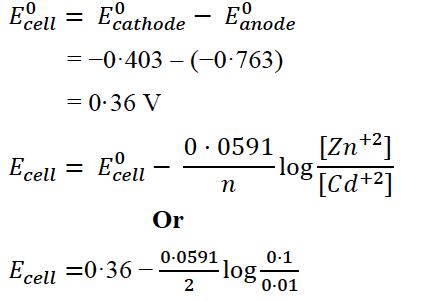𝐸cell = 0·36 − 0·0295 log 10

= 0·36 − 0·0295 ×1

= 0·3305 V

Δ𝐺o = −nFEO

= −2 × 96,500 × 0 . 36

= −69480 J /mol

= −69.48 kJ/mol

(ii)

1. Equivalent Conductivity of Electrolyte – is the conducting power of all the ions produced by dissolving one-gram equivalent in V cc. of solution.
2. Corrosion of metals– The slow and spontaneous process of the conversion of a metal into an undesirable compound (usually oxide) on exposure to atmospheric conditions is called corrosion of metals.

OR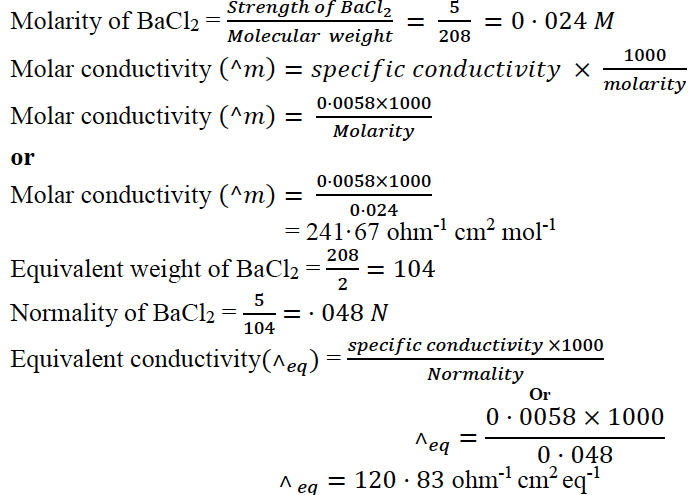When various electrode systems are arranged in the order of their Eo values, the series obtained is called electrochemical series. Elements having negative value of Eo will liberate H2 from acids.

### Question 17:

(a) (i) Explain why:

1. Nitrogen does not form pentahalides.
2. Helium is used for filling weather balloons.
3. (3) ICl is more reactive than I2.

(ii) Draw the structures of the following:

1. HClO4
2. H3PO3

OR

(b) (i) Explain why:

1. Mercury loses its meniscus in contact with ozone.
2. Halogens are coloured and the colour deepens on moving down in the group from fluorine to iodine.
3. Hydride of sulphur is a gas while hydride of oxygen is a liquid.

(ii) Complete and balance the following reactions:

(1) NaCl + MnO2 + H2SO4 → _______ + _______ + ________ + _____

(2) KMnO4 + SO2 + H2O → _______ + _______ + __________

(a) ( i)

1. Due to the absence of d-orbitals in its valence shell, nitrogen does not form pentahalides.
2. Helium being inert, non-inflammable and light gas is used in filling weather balloons.
3. I –Cl bond is weaker than I – I bond. I – Cl bond breaks easily to form halogen atoms (I and Cl)

(ii)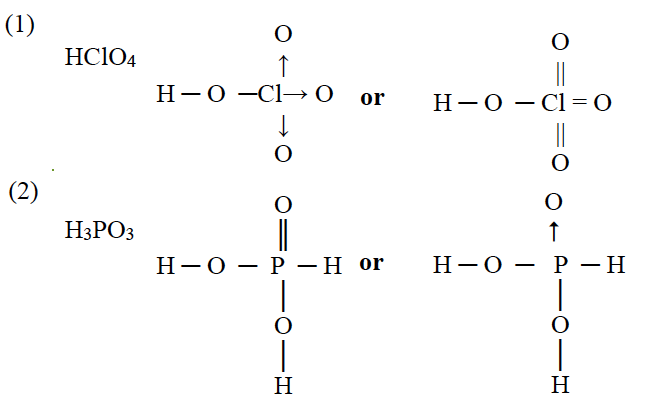OR

(b) (i)

1. Mercury in presence of ozone is oxidised to sub oxide (Mercurous oxide) which dissolves in mercury. It starts sticking to glass and loses meniscus (Tailing of Hg).

Or

2Hg + O3 → Hg2O + O2

1. Halogens are coloured due to absorption of light in visible regions. Fluorine being small absorbs violet colour and shows the complementary colour yellow whereas iodine absorbs yellow colour and shows complementary colour violet.
2. Due to the small size of oxygen atom H2O forms intermolecular H-bond and gets associated hence occurs as liquid. While H2S is a simple covalent compound hence occurs as a gas.

(ii)

1. 2NaCl + MnO2 + 3H2SO4 → 2NaHSO4 + MnSO4 + Cl2 + 2H2O

OR

2NaCl + MnO2 + 2H2SO4 → 2Na2SO4 + MnSO4 + Cl2 + 2H2O
2. 2KMnO4 + 5SO2+2H2O → K2SO4 +2MnSO4 + 2H2SO4

### Question 18:

(a) ( i ) Give balanced equations for the following reactions:

1. Benzaldehyde reacts with hydrazine.
2. Acetic acid reacts with phosphorous pentachloride.
3. Acetone reacts with sodium bisulphite.

(ii) Give one chemical test each to distinguish between the following pairs of compounds:

1. Ethanol and acetic acid
2. Acetaldehyde and benzaldehyde

OR

(b) (i) Write chemical equations to illustrate the following name reactions:

1. Clemmensen’s reduction
2. Rosenmund’s reduction
3. HVZ reaction

(ii) Explain why:

1. Acetaldehyde undergoes aldol condensation, but formaldehyde does not.
2. Acetic acid is a weaker acid as compared to formic acid.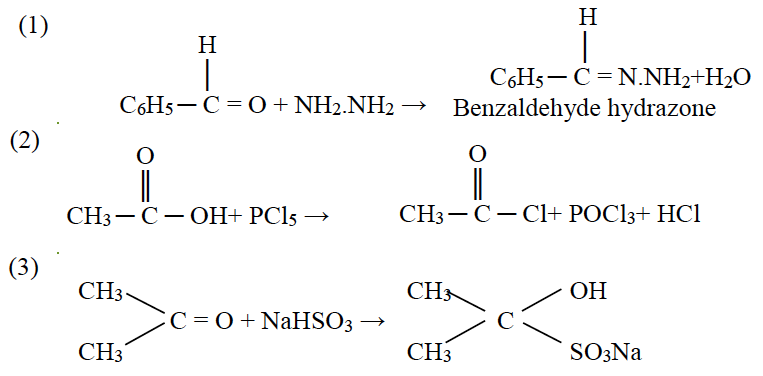(ii) (1)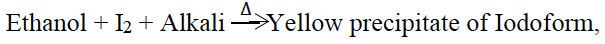acetic acid does not respond to this test.

or

Acetic acid + neutral FeCl3 → Blood red coloration,

Ethanol does not respond to this test.

(2)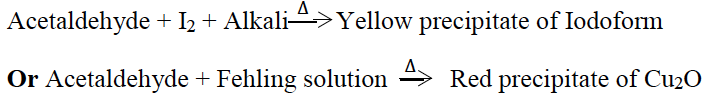Benzaldehyde does not respond to the above test.

OR

(b)(i)

1. Clemmensen’s Reduction: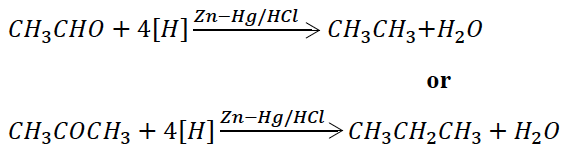1. Rosenmund’s Reduction: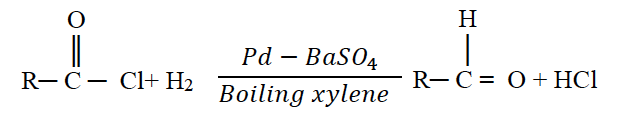1. HVZ reaction: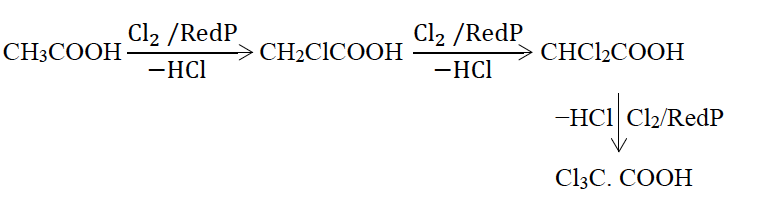(ii) (1) Aldol condensation is given by aldehydes having 𝛼–hydrogen atom. Acetaldehyde has 𝛼–H but HCHO does not have 𝛼–hydrogen atom.

Hence, does not give aldol condensation.

(2) Acetic acid has methyl (electron releasing) group which causes + I effect and decreases its acidic strength of acetic acid.

We hope students must have found ISC Class 12 Chemistry Question Paper Solution 2018 helpful for their exam preparation. We have also compiled ISC Class 12 Previous Year Question Papers for Physics, Chemistry, Maths and Biology at one place for students convenience. Happy Learning and stay tuned to BYJU’S for the latest update on ICSE/CBSE/State Boards/Competitive exams. Also, don’t forget to download the BYJU’S App.